# Mathematical ReasoningA combination of challenging content, very attractive layout, variety, significant incorporation of thinking skills, and relatively low cost merited the Mathematical Reasoning series a place among my 102 Top Picks.

This series should work very well for home educators since it works best when taught one-on-one or in small groups. The series begins at preschool level and continues through sixth grade.

While the worktexts were written to be used as either core texts or supplements, they are comprehensive enough to serve as your core texts. There are fewer problems to solve in these books than in other programs—fewer problems per page although the books are quite large. So you might supplement Mathematical Reasoning books with additional hands-on activities, games, or practice problems, and you might also use the Mathematical Reasoning Supplements I describe at the end of this review. The first three books include a suggestion that you check out the National Library of Virtual Manipulatives at http://nlvm.usu.edu/en/nav/vlibrary.html, a free website where students can work on math activities with simulated manipulatives. This site has activities up through high school level so you might also want to access it if you are using upper-level courses or any other math program for that matter.

The Mathematical Reasoning series uses a spiral approach, introducing a concept then revisiting it a number of times at intervals. Students who like variety should love this series since there are seldom two pages that look similar. Pages always have at least one illustration and are so colorful that there is little white space on a page.

As one would expect of anything from The Critical Thinking Co. (TCTC), this series emphasizes critical thinking in ways you seldom encounter in other math courses. It includes some grid-type logic puzzles (like those in the Mind Bender series from TCTC) as well as puzzles from Balance Benders and Cranium Crackers books from TCTC. Other puzzles of many types are incorporated into exercises to challenge thinking skills as well as to make it more fun.

The authors teach proper nomenclature from the beginning. For example, they use the term “line segment” rather than “line” and the term “numeral” rather than “number,” even in the preschool books.

Conceptual development is exceptionally strong since the program uses numerous ways of explaining and applying each concept. Concepts are often introduced with visual representations, sometimes representations of Base Ten Blocks or other manipulatives. You might actually use manipulatives if that is helpful for your child, but they are not required.

At the beginning of the book are very brief teaching instructions. Each lesson has directions and brief instruction on a new concept when needed. Parents and teachers might need to work with students with more explanation, examples, and practice on a new concept before expecting them to solve problems or complete activities. Even for lessons on concepts that students already understand, they might sometimes need assistance to know how to complete an unusual activity.

Answer keys are included at the back of each book from Level B (Grade 1) and up. You should not need them for the first three books.

Books are challenging and sometimes move into topics that are beyond what is typically taught at each level. Be cautious to select the correct level, and do not be concerned if your child needs to start at what appears to be a lower level than you would expect.

### Beginning 1 (Age 3)

Beginning 1 introduces the numbers 1 through 5, both visually and with numerals. It even introduces the concepts of addition and subtraction (e.g., 2 owls + 2 owls shown with pictures) at the end of the book! Other topics are size comparisons (e.g., smaller and larger), shapes, colors, identifying similar objects, counting, one-to-one correspondence, patterns, order (first, second, …fifth), measuring inches, the number line, numeral recognition, beginning logic (via Mind Bender types of puzzles), and the characteristics of triangles, squares, and rectangles.

There is a great deal of repetition in this book, and many concepts are those that children will be exposed to in normal activities around the house, so you can skip this book without missing anything critical. All concepts also show up again in Beginning 2. Some concepts in this book will be beyond many three-year-old children.

### Beginning 2 (Age 4)

Beginning 2 is similar in design to Beginning 1, but it covers numbers 0 through 13 as well as the concepts covered in Beginning 1. It starts with activities where students match numerals and groups of objects, so children should already be at least somewhat familiar with what the numbers 1 through 6 look like. Other concepts introduced in this book are the idea of zero, how to write numerals, working with a number line, visual estimation (i.e., which group appears to have more or fewer items), prepositional placement (e.g., how many dogs are in front of or beside the dog house?), dot-to-dot puzzles, right and left, and halves.

### Level A (Kindergarten)

The Beginning books emphasize counting, while Level A really moves into addition and subtraction but with sums not higher than 8 and subtraction problems with minuends (the top number) no higher than 7. Other concepts taught are odd and even numbers, patterns, counting and writing numerals up to 20, identifying similar objects, order (first, second, etc.), geometric shapes, symmetry, attributes, equations for addition and subtraction, completing bar graphs, Mind Bender type logic problems, halves and quarters (only visual concepts), coins, and time telling.

Level B introduces place value, expanded notation, counting by tens, coins, directions (including compass directions), measuring inches, the concept of measuring by other units, completing a "hundreds chart," visual analogies, transformations, bar graphs, lines of symmetry, fractions and their numerical expressions (1/2, 1/3, 1/4), thermometers, the calendar, and time telling. There are also puzzles such as dot-to-dots, Mind Bender grids, and other logic puzzles. Base Ten Blocks might be especially helpful with Level B since they are shown visually in many lessons.

Level C teaches carrying and borrowing (regrouping) up through subtraction problems with two-digit subtrahends. It teaches multiplication via skip counting, arrays, and other visual methods while it also introduces multiplication equations. Division is briefly presented at the end of the book, but it is taught only as a function opposite to multiplication. Students start learning their multiplication facts this year but shouldn’t be expected to have mastered them. Among other concepts in this level are surveys, probability, graphs, estimation, measurement, place value, odd and even numbers, metric measurement, fraction concepts, money with coins and bills, polygons, vertices, rounding numbers, writing number words, placeholders in equations, and many word and logic problems. An illustrated glossary and answer key are at the back of the book.

Level D continues with topics taught in Level C but with a heavy focus on multiplication and division up through the introduction of long division with single-digit divisors. It also teaches fraction algorithms including addition, subtraction, and multiplication of simple fractions; recognition of like and unlike denominators; and finding equivalent fractions. Among other topics new at this level are beginning work with decimals, congruent figures, using the (x,y) form to identify locations on a coordinate grid, units of measurement, rays, angles, endpoints, perimeter, area, weight, least common multiples, reflections, translations, and rotations. There are also two pages of “Time Trials” on multiplication facts.

Students do lots of work with addition, subtraction, multiplication, and division in Level E. Multiplication and division are taken to higher levels including teaching about remainders in division. While decimals receive some attention, fractions are the main topic. Among other topics added this year are factors, prime and composite numbers, mean/median/mode, inequalities, negative numbers, order of operations, working with improper fractions, and the concept of functions.

Fractions and decimals receive the most attention in Level F. Students continue to move to more challenging levels of study on concepts introduced in lower level books. New concepts are the use of a protractor, measurement of angles, elapsed time, computing a bank account balance, volume of three-dimensional objects, and geometric shapes such as hexagons and decagons.

Level G reviews concepts and skills students should have previously mastered such as addition, subtraction, multiplication, division, fractions, and decimals. While review activities include basics such as how to do regrouping for subtraction, a set of practice problems for each concept is generally accompanied by a simple puzzle that can only be solved once all problems have been answered correctly. Students encounter more challenging work with fractions and decimals as well as number properties, order of operations, measurement, geometry, and algebra. It even introduces the concepts of slope and functions. However, even the two latter concepts are introduced in a way that makes them understandable for students at this level. Mathematical puzzles such as magic squares and logic puzzles are just a few of the many critical thinking type activities built into the course.

### Mathematical Reasoning Supplements

Those who want more of a challenge for their students might want to use one of the Mathematical Reasoning Supplement books. Three are available, one for grades two through four, one for grades four through six, and one for grades seven through nine. Each book is topically arranged with lessons under headings such as Geometry, Measurement, Fractions, Patterns, and Graphing. Topics vary by level. Books are printed in black and white but they include many math illustrations such as geometric shapes and patterns. These books stretch students to apply their math skills in non-routine problems. Many of the problems have “puzzle-solving” appeal. Each book is self-contained with a complete solutions guide at the back. Student pages are reproducible for one family or class group.

Sample pages from each book as well as the table of contents may be viewed at the publisher's website.

Also see my review of Understanding Geometry, another book in this series.

### Pricing Information

When comparison prices appear, please keep in mind that they are subject to change. Click on links where available to verify price accuracy.

#### The weight-loss action diary: A journal, workbook, and motivator for permanent slenderness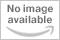#### Mathematical Reasoning Level A - Bridging the Gap Between Computation and Math Reasoning (Grade K)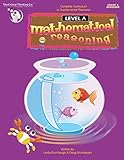#### Mathematical Reasoning Beginning 2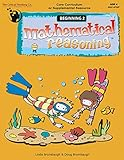#### Mathematical Reasoning Beginning 1 (PK)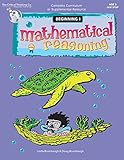#### Mathematical Reasoning Level C (Gr. 2)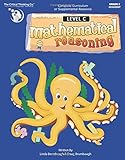#### Mathematical Reasoning Level D (Gr. 3)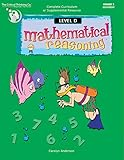#### Mathematical Reasoning Level E, Grade 4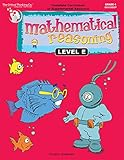#### Mathematical Reasoning Level F (Gr. 5)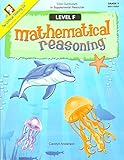#### Mathematical Reasoning Supplement - Grades 4-6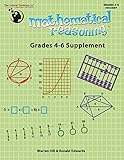#### Mathematical Reasoning: Middle School Supplement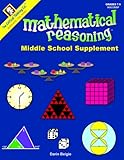#### Mathematical Reasoning Level G (Gr. 6)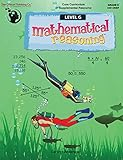Get a FREE subscription to Cathy's E-Newsletter

### My Lists

Save reviews to lists to guide curriculum choices. Register or log in to start your first list.

### Publisher's Info

Note: Publishers, authors, and service providers never pay to be reviewed. They do provide free review copies or online access to programs for review purposes.

Disclosure of Material Connection: Some of the links in the post above are "affiliate links." This means if you click on the link and purchase the item, I will receive an affiliate commission. Regardless, I only recommend products or services that I believe will add value to my readers. I am disclosing this in accordance with the Federal Trade Commission's 16 CFR, Part 255 "Guidelines Concerning the Use of Endorsements and Testimonials in Advertising."